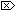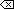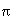The Open Group Base Specifications Issue 6
IEEE Std 1003.1-2001

#### NAME

asin, asinf, asinl - arc sine function

#### SYNOPSIS

```#include <math.h> double asin(double x); float asinf(float x); long double asinl(long double x); ```

#### DESCRIPTION

[CX]The functionality described on this reference page is aligned with the ISO C standard. Any conflict between the requirements described here and the ISO C standard is unintentional. This volume of IEEE Std 1003.1-2001 defers to the ISO C standard.These functions shall compute the principal value of the arc sine of their argument x. The value of x should be in the range [-1,1].

An application wishing to check for error situations should set errno to zero and call feclearexcept(FE_ALL_EXCEPT) before calling these functions. On return, if errno is non-zero or fetestexcept(FE_INVALID | FE_DIVBYZERO | FE_OVERFLOW | FE_UNDERFLOW) is non-zero, an error has occurred.

#### RETURN VALUE

Upon successful completion, these functions shall return the arc sine of x, in the range [-/2,/2] radians.

For finite values of x not in the range [-1,1], a domain error shall occur, and [MX]either a NaN (if supported), oran implementation-defined value shall be returned.

[MX]If x is NaN, a NaN shall be returned.

If x is ±0, x shall be returned.

If x is ±Inf, a domain error shall occur, and either a NaN (if supported), or an implementation-defined value shall be returned.

If x is subnormal, a range error may occur and x should be returned.#### ERRORS

These functions shall fail if:

Domain Error
The x argument is finite and is not in the range [-1,1], [MX]or is ±Inf.If the integer expression (math_errhandling & MATH_ERRNO) is non-zero, then errno shall be set to [EDOM]. If the integer expression (math_errhandling & MATH_ERREXCEPT) is non-zero, then the invalid floating-point exception shall be raised.

These functions may fail if:

Range Error
[MX]The value of x is subnormal.

If the integer expression (math_errhandling & MATH_ERRNO) is non-zero, then errno shall be set to [ERANGE]. If the integer expression (math_errhandling & MATH_ERREXCEPT) is non-zero, then the underflow floating-point exception shall be raised.The following sections are informative.

None.

#### APPLICATION USAGE

On error, the expressions (math_errhandling & MATH_ERRNO) and (math_errhandling & MATH_ERREXCEPT) are independent of each other, but at least one of them must be non-zero.

None.

#### FUTURE DIRECTIONS

None.

feclearexcept() , fetestexcept() , isnan() , sin() , the Base Definitions volume of IEEE Std 1003.1-2001, Section 4.18, Treatment of Error Conditions for Mathematical Functions, <math.h>

#### CHANGE HISTORY

First released in Issue 1. Derived from Issue 1 of the SVID.

#### Issue 5

The DESCRIPTION is updated to indicate how an application should check for an error. This text was previously published in the APPLICATION USAGE section.

#### Issue 6

The asinf() and asinl() functions are added for alignment with the ISO/IEC 9899:1999 standard.

The DESCRIPTION, RETURN VALUE, ERRORS, and APPLICATION USAGE sections are revised to align with the ISO/IEC 9899:1999 standard.

IEC 60559:1989 standard floating-point extensions over the ISO/IEC 9899:1999 standard are marked.

End of informative text.

UNIX ® is a registered Trademark of The Open Group.
POSIX ® is a registered Trademark of The IEEE.
[ Main Index | XBD | XCU | XSH | XRAT ]